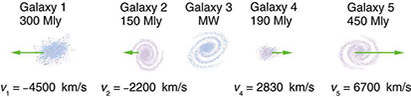# Notes

## Solution:

The weight of an object is computed using the formula$\displaystyle \text{Weight}=\text{mass}\times 9.81\:\text{m/s}^2$

### Part A$\displaystyle \text{Weight}=8\:\text{kg}\times 9.81\:\text{m/s}^2$$\displaystyle \text{Weight}=78.5\:\text{newtons}$          ◀

### Part B$\displaystyle \text{Weight}=0.04\:\text{kg}\times 9.81\:\text{m/s}^2$$\displaystyle \text{Weight}=0.3924\:\text{newtons}$          ◀

### Part C$\displaystyle \text{Weight}=760\:\text{Mg}\times \frac{1000\:\text{kg}}{1\:\text{Mg}}\times 9.81\:\text{m/s}^2$$\displaystyle \text{Weight}=7\:455\:600\:\text{newtons}$          ◀

## Solution:

#### Solution will be posted soon.

We already have the answer to the problem, but we need some time to encode it. For the mean time, you can request the solution to the problem to expedite the posting of solution. Also, you can purchase the complete solution manual of College Physics by Openstax.

## Solution:

#### Solution will be posted soon.

We already have the answer to the problem, but we need some time to encode it. For the mean time, you can request the solution to the problem to expedite the posting of solution. Also, you can purchase the complete solution manual of College Physics by Openstax.

## Solution:

#### Solution will be posted soon.

We already have the answer to the problem, but we need some time to encode it. For the mean time, you can request the solution to the problem to expedite the posting of solution. Also, you can purchase the complete solution manual of College Physics by Openstax.

## College Physics 3.67 – The angle of a puck’s velocity relative to the player

#### An ice hockey player is moving at 8.00 m/s when he hits the puck toward the goal. The speed of the puck relative to the player is 29.0 m/s. The line between the center of the goal and the player makes a 90.0º angle relative to his path as shown in Figure 3.63. What angle must the puck’s velocity make relative to the player (in his frame of reference) to hit the center of the goal?Figure 3.63 An ice hockey player moving across the rink must shoot backward to give the puck a velocity toward the goal.

## Solution:

#### Solution will be posted soon.

We already have the answer to the problem, but we need some time to encode it. For the mean time, you can request the solution to the problem to expedite the posting of solution. Also, you can purchase the complete solution manual of College Physics by Openstax.

## Solution:

#### Solution will be posted soon.

We already have the answer to the problem, but we need some time to encode it. For the mean time, you can request the solution to the problem to expedite the posting of solution. Also, you can purchase the complete solution manual of College Physics by Openstax.

## Solution:

#### Solution will be posted soon.

We already have the answer to the problem, but we need some time to encode it. For the mean time, you can request the solution to the problem to expedite the posting of solution. Also, you can purchase the complete solution manual of College Physics by Openstax.

## Solution:

#### Solution will be posted soon.

We already have the answer to the problem, but we need some time to encode it. For the mean time, you can request the solution to the problem to expedite the posting of solution. Also, you can purchase the complete solution manual of College Physics by Openstax.

## College Physics 3.63 – Velocities relative to the galaxies

#### The great astronomer Edwin Hubble discovered that all distant galaxies are receding from our Milky Way Galaxy with velocities proportional to their distances. It appears to an observer on the Earth that we are at the center of an expanding universe. Figure 3.62 illustrates this for five galaxies lying along a straight line, with the Milky Way Galaxy at the center. Using the data from the figure, calculate the velocities: (a) relative to galaxy 2 and (b) relative to galaxy 5. The results mean that observers on all galaxies will see themselves at the center of the expanding universe, and they would likely be aware of relative velocities, concluding that it is not possible to locate the center of expansion with the given information.Figure 3.62 Five galaxies on a straight line, showing their distances and velocities relative to the Milky Way (MW) Galaxy. The distances are in millions of light years (Mly), where a light year is the distance light travels in one year. The velocities are nearly proportional to the distances. The sizes of the galaxies are greatly exaggerated; an average galaxy is about 0.1 Mly across.

## Solution:

#### Solution will be posted soon.

We already have the answer to the problem, but we need some time to encode it. For the mean time, you can request the solution to the problem to expedite the posting of solution. Also, you can purchase the complete solution manual of College Physics by Openstax.

## Solution:

#### Solution will be posted soon.

We already have the answer to the problem, but we need some time to encode it. For the mean time, you can request the solution to the problem to expedite the posting of solution. Also, you can purchase the complete solution manual of College Physics by Openstax.